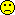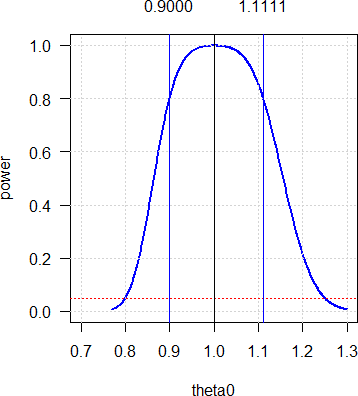## Symmetrical in log-scale [Power / Sample Size]

Hi BE-proff,

» No, I didn't catch an idea.» Do you think that absence of symmetry critical?

Power curves are asymmetrical around 1 in linear scale (as is the acceptance range) but symmetrical around 0 in logarithmic scale (AR: ±0.2231). Try this (CV 0.26, GMR 0.9, n 60 for target power 80%):

library(PowerTOST) CV     <- 0.26 GMR    <- 0.90 theta0 <- seq(1/1.3, 1.3, length.out=501) n      <- sampleN.TOST(CV=0.26, theta0=GMR, print=FALSE)[["Sample size"]] power  <- numeric(length(theta0)) for (j in seq_along(theta0)) {   power[j] <- power.TOST(CV=CV, theta0=theta0[j], n=n) } dev.new(record=TRUE) op     <- par(no.readonly=TRUE) par(pty="s", ask=TRUE) # linear scale # plot(theta0, power, type="l", las=1, col="blue", lwd=2,      xlim=c(2-max(theta0), max(theta0)), ylim=c(0, 1)) grid() abline(h=0.05, col="red", lty="dotted") abline(v=c(1, GMR, 1/GMR), col=c("black", rep("blue", 2))) mtext(sprintf("%.4f", c(GMR, 1/GMR)), side=3,       at=c(GMR, 1/GMR), line=1) # logarithmic scale # plot(log(theta0), power, type="l", las=1, col="blue", lwd=2,      xlim=c(-1, +1)*max(abs(range(log(theta0)))), ylim=c(0, 1)) abline(h=0.05, col="red", lty="dotted") grid() abline(h=0.05, col="red", lty="dotted") abline(v=log(c(1, GMR, 1/GMR)), col=c("black", rep("blue", 2))) mtext(sprintf("%+.4f", log(c(GMR, 1/GMR))), side=3,       at=log(c(GMR, 1/GMR)), line=1) par(op)

Press or click in the graphics window to go to the next plot. To switch between plots navigate to the graphics window and use / to switch plots.The power curve is positively skewed. For any –∆ you get the same power at 1/∆. In the example: For GMR 1.1111 as for GMR 0.90 since 1/0.90 = 1.1111. In many protocols I read “planned for a T/R-ratio of 0.90 to 1.10”. Nope. If the sample size estimation was done for 0.90 it covers anything up to 1.1111. Or the other way ’round: If it was done for 1.10 it would cover anything down to only 1/1.1 = 0.9091 (and not 0.90). Hence, the latter is stupid – unless you know (!) that the GMR will be >1. If you don’t know the sign of ∆, always plan for a GMR <1 and you will be on the safe side.

The second part of the script demonstrates that in log-scale (where the entire analysis is done) everything is symmetrical indeed:Hope that helps.

Cheers,
Helmut SchützThe quality of responses received is directly proportional to the quality of the question asked. 🚮
Science QuotesIng. Helmut Schütz